# Sulfur difluoride (SF2) lewis dot structure, molecular geometry, bond angle, polar or nonpolar

Home  > Chemistry Article > SF2 lewis structure and its molecular geometry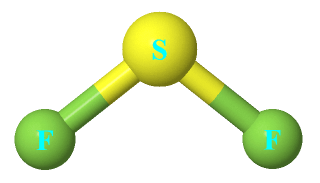Sulfur difluoride has a molar mass of 70.062 g/mol, it is highly unstable. It is an inorganic compound with the chemical formula SF2.

In this article, we will discuss Sulfur difluoride (SF2) lewis structure, molecular geometry, bond angle, polar or nonpolar, its hybridization, etc.

Sulfur difluoride is formed by the reaction between one mole of sulfur dichloride with 2 moles of potassium fluoride.

⇒ SCl2 + 2 KF → SF2 + 2 KCl

 Name of Molecule Sulfur difluoride Chemical formula SF2 Molecular geometry of SF2 Bent Electron geometry of SF2 Tetrahedral Hybridization Sp3 Nature Polar molecule Bond angle 98º Total Valence electron for SF2 20
Page Contents

## How to draw lewis structure of SF2?

SF2 Lewis structure is made up of two atoms, sulfur, and fluorine, the sulfur is in the central position and fluorine atoms are on either side of it. The lewis structure of SF2 contains 16 nonbonding electrons and 4 bonding electrons.

The lewis structure of SF2 is similar to the SBr2 and SCl2.

Here let’s see how to draw it.

## Follow some steps for drawing the lewis dot structure for SF2

1. Count total valence electron in SF2

In the very first step, we have to count the number of valence electrons available for drawing the Lewis structure of SF2. For this, we have to count the valence electrons in sulfur and fluorine atoms.

The best way to determine the valence electron in an atom is by looking at the group number in the periodic table.

As sulfur atom belongs to group 16th in the periodic table and fluorine is situated in 17th group, hence, the valence electron for sulfur is 6 and for fluorine atom, it is 7.

⇒ Total number of the valence electrons in sulfur = 6

⇒ Total number of the valence electrons in fluorine = 7

∴ Total number of valence electron available for the SF2 Lewis structure = 6 + 7(2) = 20 valence electrons         [∴SF2 molecule has one sulfur and two fluorine atoms]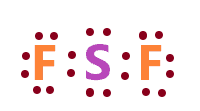2. Find the least electronegative atom and place it at center

An atom with a less electronegative value is more preferable for the central position in the lewis diagram because they are more prone to share the electrons with surrounding atoms.

So, in the Sf2 molecule, the electronegativity of sulfur is 2.58 and the electronegativity of fluorine is 3.98. Clearly, sulfur is a less electronegative atom.

So, just put the sulfur in the center position and both fluorine atoms on either side of it.3. Connect outer atoms to central atom with a single bond

In the third step, draw a single bond to connect both outer atoms (fluorine) to the central atom(sulfur).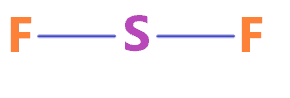As in the shown figure, we draw the single bond from the left and right side fluorine atoms to the central atom. Now count the valence used in the above structure.

A single bond contains two electrons, and, in the above structure, two single bonds are used, hence, 4 valence electrons are used in the above structure from the total of 20 valence electrons that is available for drawing the Lewis structure of SF2.

∴ (20 – 4) = 16 valence electrons

So, we are left with 16 valence electrons more.

4. Place remaining electrons on outer atoms and complete their octet

In this step, we have to complete the octet of outer atoms first, the octet means having 8 electrons in the valence shell of an atom.

Now in the SF2 molecule, the fluorine is the outer atom and it needs 8 electrons in its valence shell to complete its octet.

So, just start putting the remaining valence electron on fluorine atoms till they complete their octet.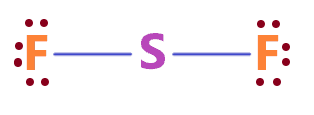So, both fluorine atoms in the above structure completed their octet, because both of them have 8 electrons(6 electrons represented as dots + 2 electrons in a single bond) in their valence shell.

Now again count the total valence electrons used in the above structure.

In the above structure, there are 12 electrons represented as dots + 2 single bonds means 4 electrons are used.

Hence, (12 + 4) = 16 total electrons are used in the above structure from a total of available 20 valence electrons.

Therefore, we are left with 4 more valence electrons.

5. Complete the central atom octet and make multiple bonds if necessary

As we already completed the octet of both fluorine atoms, now we have to complete the octet of the central atom which is sulfur in the SF2 molecule.

In the 4th step structure, we see that the sulfur central atom attached with 2 single bonds means, it has already 4 electrons in its valence shell. Hence, sulfur needs only 4 more electrons to complete its octet

We already have 4 remaining valence electrons, hence, put these remaining electrons around the sulfur atom and complete its octet.### SF2 lewis structure

That’s all, we have used all the valence electrons available for drawing the Lewis structure of SF2 and all the atoms in the above structure completed their octet as all of them have 8 electrons in their valence shell.

Now just check the formal charge for the above SF2 lewis structure.

6. Check the stability with the help of a formal charge concept

The lesser the formal charge on atoms, the better is the stability of the lewis diagram.

To calculate the formal charge on an atom. Use the formula given below-

⇒ Formal charge = (valence electrons – lone pair electrons –  1/2 bonding electrons)

Let’s count the formal charge on the fluorine atom first, both fluorine atoms in the SF2 Lewis structure(5th step) have the same bonded pair and lone pair, so, just count the F.C. for the one fluorine atom.

For fluorine atom:

⇒ Valence electrons of fluorine = 7

⇒ Lone pair electrons on fluorine= 6

⇒ Bonding electrons around fluorine(1 single bond) = 2

∴ (7 – 6 – 2/2) = 0 formal charge on both fluorine atoms.

For sulfur atom

⇒ Valence electrons of sulfur = 6

⇒ Lone pair electrons on sulfur = 4

⇒ Bonding electrons around sulfur (2 single bonds) = 4

∴ (6 – 4 – 4/2) = 0 formal charge on the sulfur central atom.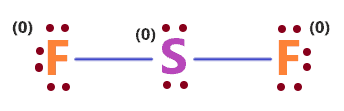So, this is our most stable and appropriate lewis dot structure or electron dot structure of SF2.

## What are the electron and molecular geometry of SF2?

The molecular geometry of SF2 is Bent because the central atom sulfur is attached with two bond pairs and there are two lone pairs on it, so, according to the VSEPR theory, these bond pairs and lone pair around the sulfur will repel each other, as a result, they will be pushed apart and pushes down the bonded atoms, giving the shape of SF2 similar to the bent.

Since the central atom in SF2 has four regions of electron density(2 bond pairs + 2 lone pairs), its molecular geometry will be bent and its electron geometry will be tetrahedral.### SF2 molecular geometry

Also, the generic formula for SF2 is AX2N2 according to the VSEPR theory and its chart.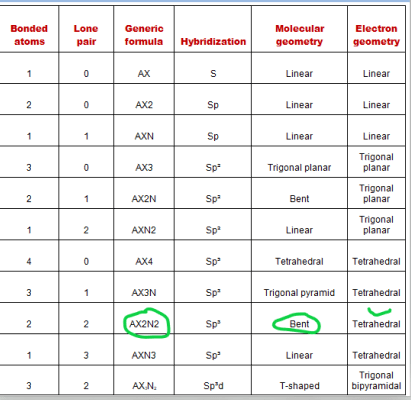## Hybridization of SF2

Let’s find the hybridization of SF2 through the steric number of its central atom.

“Steric number is the addition of a total number of bonded atoms around a central atom and the lone pair present on it.”

∴ Steric number of SF2 = (Number of bonded atoms attached to sulfur + Lone pair on sulfur)

As per the lewis structure of SF2, the sulfur atom is bonded with two fluorine atoms and it contains two lone pairs of electrons.

∴ Steric number of SF2 = (2 + 2) = 4

 Steric number Hybridization 1 S 2 Sp 3 Sp² 4 Sp³ 5 Sp³d 6 Sp³d²

So, for a steric number of four, we get the Sp3 hybridization on the sulfur atom in the SF2 molecule.

## The bond angle of SF2

The bond angle of SF2 is around 98º as due to the lone pair on the sulfur central atom, both fluorine atom pushes down in the downward direction that contract the angle between them, and gives the approx F-S-F 98º angle.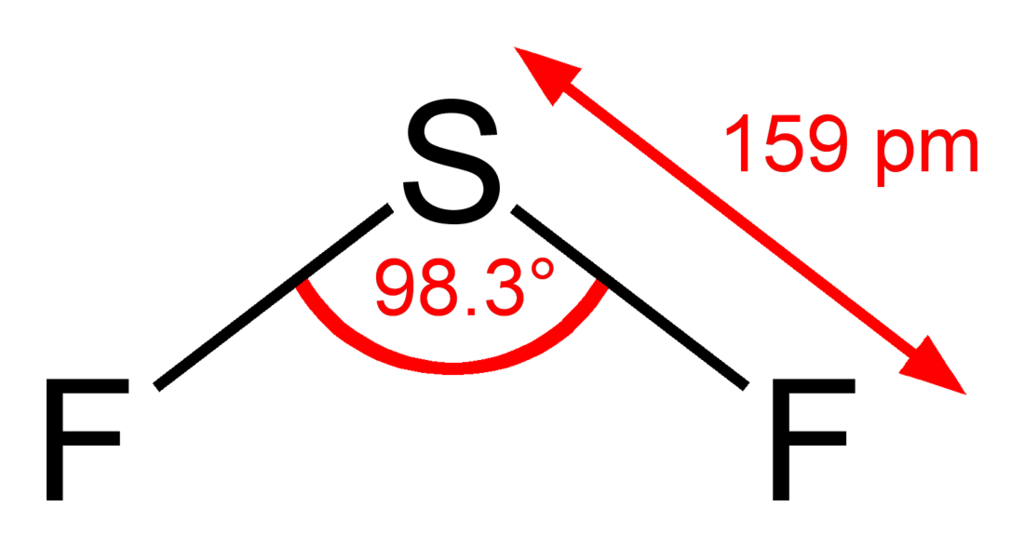## Sulfur difluoride polarity: is SF2 polar or nonpolar?

Is SF2 polar or non-polar? SF2 is polar because of its bent geometrical shape caused by the lone pair on the central atom which pushes down the bonded atoms to keep the repulsive force minimal, all this, distorted the shape of the molecule and causes uneven charges distribution, this result in a non-zero dipole moment and makes the SF2 polar in nature.

⇒ The electronegativity of fluorine = 3.98

⇒ The electronegativity of sulfur = 2.58

∴ The difference of electronegativity between sulfur and fluorine = 1.4

According to the Pauling scale, the S-F bond is highly polar since the electronegativity difference between them is way more than 0.5.

Since fluorine is more electronegative than the sulfur atom, the fluorine tries to attract the more shared bonded electrons towards its side and this will induce a negative charge on it. Whereas the sulfur atom will get a positive charge

Because of this separation of charge, there will be a dipole in between the sulfur and fluorine, and these dipole moments will not be canceled out because of the bent shape(asymmetrical) of the SF2 molecule, and this makes it polar in nature.

## FAQ

### How many bonding pairs and lone pairs are present in the lewis structure of SF2?

Bonding pairs are the pair of electrons that are in a bond. A single bond has one bond pair means 2 bonding electrons.

Lone pairs are those represented as dots in the lewis diagram that do not take part in the formation of bonds and are also called nonbonding electrons.

So, in the SF2 lewis structure, there are 2 bond pairs means 4 bonding electrons and 8 lone pairs (2 on central atom + 3 on each fluorine atom) are present.

### Why the molecular geometry of SF2 is bent but its electron geometry is tetrahedral?

The electron geometry considers both bond pair and lone pair while predicting the geometry of the molecule. And the molecular geometry only considers the bond pair to predict the shape of the molecule.

So, as per the SF2 lewis structure, the sulfur central atom is surrounded by 4 electron pairs(2 bond pairs + 2 lone pairs).

∴ Four regions of electron density always form a tetrahedral geometry, hence, the electron geometry of SBr2 is tetrahedral.

## Summary

• The total valence electron is available for drawing the SF2 Lewis structure is 20.
• The molecular geometry of SF2 is bent and its electron geometry is tetrahedral.
• The lewis structure of SF2 has 4 bonding electrons and 16 nonbonding electrons.
• The bond angle of SF2 is around 98º and its hybridization is Sp3.
• SF2 is polar because of its bent geometrical shape and large electronegativity difference between sulfur and a fluorine atom.
##### Subscribe to Blog via Email

Join 2 other subscribers

Share it...